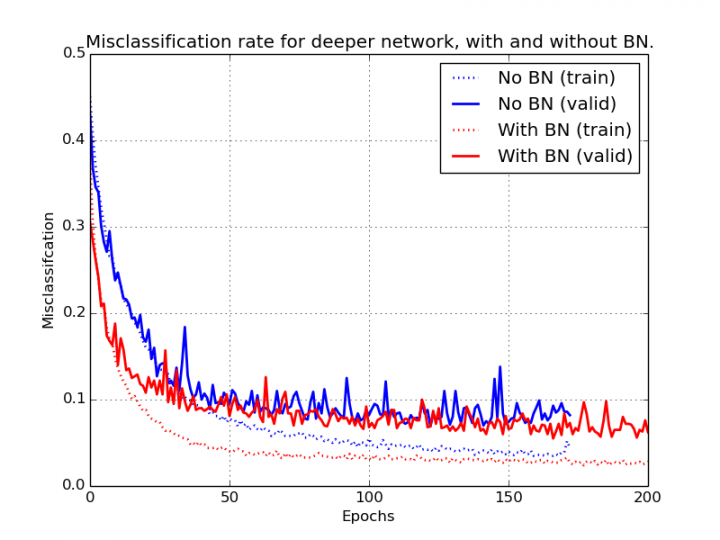# Regularization Techniques — a list down

Source: Deep Learning on Medium# Regularization Techniques — a list down

## General Knowledge

Regularization techniques are used to reduce the overfitting in the learning process. Overfitting occurs when our model learns the input so much better that it could only further resolve the scenarios that seem similar to the input. It behaves very badly if something gets out of the scope of the input. Overfitting causes a lot of problems if we have our overfitted model in the production. Let’s learn the techniques.

One way to reduce overfitting is to reduce the number of parameters — that’s a conventional approach. Now, instead of reducing the number of parameters, we use the all the parameters, but we penalize the non-required, unimportant parameters which do not affect the output. We penalize those parameters for being non-zero, for their existence — that’s an unconventional approach.

# L2 Regularization

L1 and L2 regularization are two different ways to represent the same thing.

As per the L2 regularization, the thing that matters is a loss, and if the loss in output will be more, we will backpropagate more to decrease the parameter, aka parameters to decrease the loss. In this procedure, weights which would non-zero or slightly near to zero would tend to become zero, thus, ineffective. This is how L2 regularization occurs.

Thus, we add the sum of the square of end weights multiplied with the number `a` to the the loss. This number is also known as `weight decay.`

# L1 Regularization

L1 regularization is also known as weight decay. As per this regularization technique, instead of adding something to weights, we subtract directly from the gradients. Now, what is subtracted from the gradients is the loss derivative, as defined in the above image. So, we are doing the same thing, if the loss would be higher; still, backpropagation would occur, and our weights would be improved. And if we directly subtract from the gradients something, that would also enhance the weights.